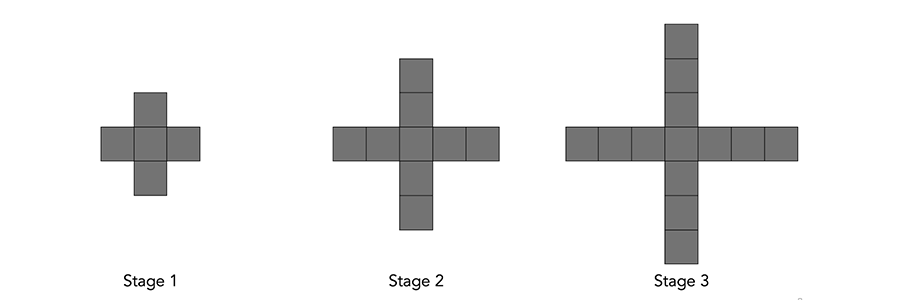# Math Problems

## Problem of the Month - March 2023

You are ordering round pizzas.

If you want more pizza, would it make sense to order two 12 inch diameter pizzas or one 18 inch diameter pizza?

Math Problems

## February 2023 - Problem of the Month

This Month’s Problem

Consider the following pattern.Math Problems

## January 2023 - Problem of the Month

In the puzzle below, the value of a square in any of the top three levels is the sum of the two squares directly beneath it.  What will be the value in the top square?

Math Problems

## December 2022 - Problem of the Month

How many squares do you see in this picture?  (There are more than 20)

There are 30 squares.  What if instead of a 4x4 grid, we started with a 5x5 grid.  How many squares would there be?Math Problems

## November 2022 - Problem of the Month

74 means 7x7x7x7 which is equal to 2401 so when 74 is expressed as an integer, the last digit is a 1.

What is the last digit of 711 when it is expressed as an integer?

Solution

The last digit is a 3.  What is the last digit of 7200 when it is expressed as an integer?

Math Problems

## October 2022 - Math Problem

This Month’s Problem

Consider this list of numbers

1, 1, 1, 4, 4, 4, 7, 7, 7, 10, 10, 10

Pick any 3 numbers from the list and add them together.

Repeat the process with a new set of numbers from the original list.

What do you notice about the sums?

Solution

The sum is always a multiple of 3.

Can you increase the list by six numbers so that selecting three of them will always result in a sum that is a multiple of 3?

Math Problems

## June 2022 - Math Problem

Use this Scratch program to play "High-Low".  Click on the green flag to start.

Try to come up with a strategy to minimize the number of guesses that it takes to come up with the number.

Math Problems

## May 2022 - Math Problem

The number 15 can be written as the sum of consecutive whole numbers in three ways.

15 = 7 + 8

15 = 4 + 5 + 6

15 = 1 + 2 + 3 + 4 + 5

In how many ways can you write 105 as the sum of whole numbers?

Solution:

Here are five ways:

105 = 52 + 53

105 = 34 + 35 + 36

105 = 19 + 20 + 21 + 22 + 23

105 = 12 + 13 + 14 + 15 + 16 + 17 + 18

105 = 1 + 2 + 3 + 4 + 5 + 6 + 7 + 8 + 9 + 10 + 11 + 12 + 13 + 14

In how many ways can you write 24 as the sum of whole numbers?

Math Problems

## April 2022 - Math Problem

Create a set of 9 cards that are numbered 1 to 9.  Select 4 cards at random and use the numbers on all  4 the cards and the 4 operations (add, subtract, multiply and divide) so that the result is 24.  For example if the numbers are 9, 6, 8, 1 then one possibility is 1 x (9 - 6) x 8.

What if the numbers were 6, 2, 7, 3?

You can also play the interactive game online.

Solution:

One possibility is 7 x 6 / 2 + 3

What if the numbers were 9, 3, 7, 7?

Math Problems